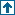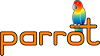parrotcode: The Parrot Debugger Contents | Documentation

# NAMEdocs/debugger.pod - The Parrot Debugger

# ABSTRACTThis document describes pdb, the Parrot Debugger.

# DESCRIPTIONStarting from version 0.0.6 Parrot has its own debugger, which is modeled after Perl's one. Its name is pdb, and is an interactive environment that let you step through bytecode, set breakpoints, evaluate assembly instructions and peek at the interpreter status.

A good (well, at least some) knowledge of the Parrot internals is obviously required to read this document. Some familiarity with debugging principles is also mandatory beyond this point.

# BUILDING pdbThe debugger is not built with Parrot, but you should make it with its specific target:

`  make pdb`

(where `make` is the same `make` incarnation you used to build Parrot).

If everything goes well, you should come up with a pdb executable in the same directory as the Parrot program.

# THE DEBUGGER SHELLTo start the debugger type:

`  pdb file.pbc`

That is, pdb takes exactly one argument, which is the Parrot bytecode that you're going to debug. pdb will automatically load and disassemble the bytecode file for you.

Note that you can't pass command line arguments to your program when you invoke the debugger. See the `run (r)` command below for this.

After the version banner, you'll see the friendly debugger prompt:

`  (pdb)`

pdb is ready to receive commands and give output. To list the available commands type 'h'. To quit the debugger type 'q'.

As with the Perl debugger, whenever it halts and shows you a line of code, it is always the line it's about to execute, not the one that it has just executed.

# DEBUGGER COMMANDSAlways remember that you can enter 'h' to get a list of commands (this document may be outdated in respect to the actual debugger, so let it speak for itself).

Most commands can be shortened to their first letter. When available, this is signaled by the letter in parentheses after the command name Thus, `help (h)` means that the command can be given as 'help' or just 'h'. On the other hand, `load` can only be given as 'load', verbatim. And the debugger is case sensitive.

A blank line always repeats the last command entered.

Also note that at this point in its development, pdb has very poor error checking on commands and their arguments, so type carefully or something bad will happen. Feel free to report bugs, or better yet patch the source code (see "FILES" below).

disassemble

Disassemble a loaded bytecode file. This will turn a file loaded with `load` into proper Parrot assembler.

Load a source code (assembler) file. The syntax is:

`  load FILE`
list (l)

List the source code. The syntax is:

`  list [FROM] [NUM]`
Both arguments are optional. By default `FROM` is from where the last list command ended (or the first line if this is the first invocation) and `NUM` is 10. That is, it lists the source code ten lines at a time.

Note that the disassembled source code is not the same as the original source code: labels take the names `L1 .. Ln` and opcodes are fully qualified (eg. `set_i_ic` instead of just `set`). See also `eval (e)`.

Example:

```  # lists the first three source code lines
(pdb) l 1 3
1  set_i_ic I1,0
2  L3:  print_sc "fact of "
3  print_i I1```
run (r)

Run (or restart) the program. The syntax is:

`  run [ARGUMENTS]`
Any arguments you give are passed as command line arguments to the program (ie. they populate P0).

After the program has ended, you can run it again with this command. See also the `continue (c)` command.

Example:

```  (pdb) r
Restarting
fact of 0 is: 1
fact of 1 is: 1
fact of 2 is: 2
fact of 3 is: 6
fact of 4 is: 24
fact of 5 is: 120
fact of 6 is: 720
Program exited.```
break (b)

Add a breakpoint. The syntax is:

`  b LINE [if CONDITION]`
If you want a conditional breakpoint you should first specify the register that is involved in the condition (at least one must be), the comparison and then the third argument can be either another register or a constant, which must be of the same type as the first register specified.

The command returns a number which is the breakpoint identifier. You should note this number for the `delete (d)` command (see below).

Example:

```  # sets a breakpoint on line 10 (will be breakpoint 0)
(pdb) b 10
Breakpoint 0 at line 10

# another breakpoint on line 11 (will be breakpoint 1)
(pdb) b 11
Breakpoint 1 at line 11

# break at line 4 if I16 is less than or equal to 123456
(pdb) b 4 if I16 <= 123456
Breakpoint 2 at line 4

# break at line 4 if N27 is greater than 5.23
(pdb) b 5 if N27 > 5.23
Breakpoint 3 at line 5

# break at line 4 if S2 is equal to S13
(pdb) b 6 if S2 == S13
Breakpoint 4 at line 6

# break at line 4 if S5 is equal to "stop"
(pdb) b 7 if S2 == "stop"
Breakpoint 5 at line 7```
watch (w)

Add a watchpoint. The syntax is:

`  w CONDITION`
The condition has the same format as in `break`

delete (d)

Delete a breakpoint. The syntax is:

`  d NUM`
The `NUM` argument is the breakpoint number (from 0 to N) as emitted by the `break (b)` command. It is NOT the line that has the breakpoint.

Example:

```  # delete the first breakpoint (was on line 10, see example above)
(pdb) d 0```
disable

Disable a breakpoint. The syntax is the same as for the `delete` command. Disabled breakpoints can be reenabled with `enable`.

enable

Reenable a disabled breakpoint. The syntax is:

`  enable [NUM]`
where `NUM` is the number of the breakpoint.

continue (c)

Continue the program execution. The syntax of this command is:

`  continue [NUM]`
Without arguments, the command just runs the source code until a breakpoint is found (or until the end of the program).

If you specify a number, it will skip the next `NUM` breakpoints it encounters.

When the program has ended, continue will do nothing. Use `run (r)` to execute it again.

next (n)

Run the next instruction. The syntax is:

`  next [NUM]`
`NUM` defaults to 1, but you can give a number of instructions to execute before stopping again.

eval (e)

Run an instruction. The syntax is:

`  eval INSTRUCTION`
Example:

```  (pdb) e set I0, 42

(pdb) e print I0
42

(pdb) p i
I0 =           42
I1 =            0
...```
trace (t)

Trace the next instruction. The syntax is:

`  trace [NUM]`
It executes the next `NUM` instructions (default is 1) just as `next (n)` does, but printing additional trace information. This is the same as the information you get when running Parrot with the `-t` option.

Example:

```  # executes 2 instructions and trace them
(pdb) t 2
PC=0; OP=67 (set_i_ic); ARGS=(I1=0, 0)
PC=3; OP=24 (print_sc); ARGS=("fact of ")
fact of
3  print_i I1```
print (p)

Print the interpreter registers. The syntax is:

`  print VALUE`
`VALUE` may be:

A register name: `I3`

Prints out the single register specified.

A register type: `i`, `n`, `s`, or `p`

Prints out all registers of the given type

An aggregate key: `P0`

Looks up the given (integer- or string-valued) key in a PMC register.

For PMC registers, the command will print the number, the class of the PMC (in square brackets) and its string representation (when available). It prints <null pmc> for uninitialized PMC registers.

Example:

```  # prints the content of I2
(pdb) p i2
Integer Registers:
I2 =              0

# prints the content of P0
(pdb) p P0
PMC Registers:
P0 = [PerlArray]

# prints the content of all string registers
(pdb) p s
String Registers:
0 =
Buflen  =                  4
Flags   =                  0
Bufused =                  4
Strlen  =                  4
Offset  =                  0
String  =       Just
1 =
Buflen  =                  8
Flags   =                  0
Bufused =                  7
Strlen  =                  7
String  =       another
2 =
Buflen  =                  8
Flags   =                  0
Bufused =                  6
Strlen  =                  6
String  =       Parrot
3 =
Buflen  =                  8
Flags   =                  0
Bufused =                  6
Strlen  =                  6
String  =       hacker
4 =
5 =
6 =
7 =
8 =
# ... and so on```
stack (s)

Examine the stack. The syntax is:

```  stack TYPE [NUM]
stack NUM```
The first form prints entries from the register stacks (eg. results from `push_i`, `push_n`, `push_s` and `push_p`). For the meaning of `TYPE` see the `print (p)` command. The `NUM` argument is the frame number (defaults to 0). The whole frame (0..31) is printed out.

The second form prints a single entry (`NUM`) from the generic stack (eg. results from `save`), prepending the type of the entry on output.

Example:

```  # prints the value on top of the generic stack
(pdb) s 0
Integer =             42

# prints the first integer register stack frame
(pdb) s i
Integer stack, frame 0, depth 0
Integer Registers:
0 =              0
1 =              0
2 =              0
3 =              0
4 =              0
5 =              0
6 =              0
7 =              0
8 =              0
# ... and so on```
info

Print interpreter information.

Example:

```  (pdb) info
Total memory allocated = 81936
DOD runs = 6
Collect runs = 0
Active PMCs = 8197
Active buffers = 7
Total PMCs = 21840
Total buffers = 48
Header allocations since last collect = 0
Memory allocations since last collect = 2```
quit (q)

Exit the debugger.

help (h)

Prints information about debugger commands. The syntax is:

`  help [COMMAND]`
If `COMMAND` is omitted, prints a list of the available commands.

# FILESsrc/pdb.c

This is the file that will produce the executable. Nothing fancy here, only the `main` function.

src/debug.c

Most of the debugger is implemented here. You may want to start from the `PDB_run_command` function and go down from there for the real meat.

src/embed.c

`Parrot_debug`, the function which launches the debugger, is implemented here.

include/parrot/debug.h

This defines all the PDB structures, which hold data used by the debugger.

# HISTORYVersion 1.0

First version (CVS debug.c revision 1.24), authored by Aldo Calpini